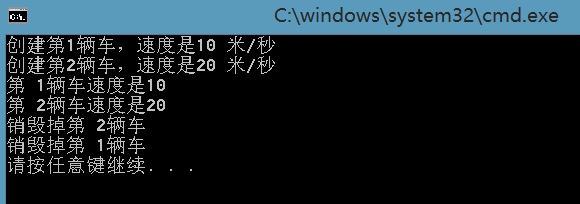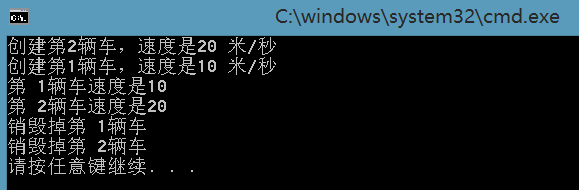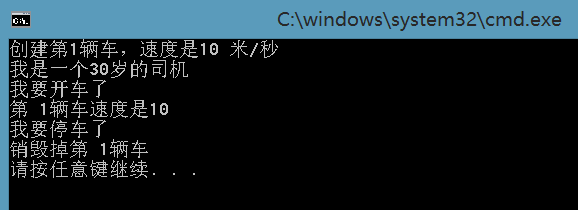# C++多个类中构造函数与析构函数的调用顺序

在C++中有一个很重要的法则：使用构造函数创建对象的顺序与使用析构函数释放对象的顺序相反。对于一些C++的初学者来说，这是一条有点费解的法则，那么该怎么理解和清晰的表现出来呢？下面我们通过程序来体现一下：

#include<iostream>
using namespace std;

//创建一个汽车类
class Car
{
public:
//构造函数
Car(short, int);
//析构函数
~Car();
void move();
private:
short speed;
int num;
};

Car::Car(short s, int n)
{
speed = s;
num = n;
cout << "创建第" << num << "辆车，速度是" << speed << " 米/秒" << endl;
}

Car::~Car()
{
cout << "销毁掉第 " << num << "辆车" << endl;
}

void Car::move()
{
cout << "第 " << num << "辆车速度是" << speed << endl;
}

//主函数
void main()
{
//先创建第car1对象，再创建car2对象
Car car1(10, 1), car2(20, 2);
car1.move();
car2.move();
}

编译执行的结果如下(编译环境为Visual Studio 2013)：分析输出结果，我们可以看到，在主函数中是首先创建了car1类，后创建了car2类，所以在执行构造函数的顺序上，是先执行car1对象的构造函数，后执行car2对象的构造函数。而在主函数执行结束，要调用析构函数的时候，根据开始说的法则，则会先执行car2类的析构函数，后执行car1类的析构函数。这就是“使用构造函数创建对象的顺序与使用析构函数释放对象的顺序相反”这条法则的一个很好的体现

那么我们可以举一反三，如果想先执行car1类的析构函数，后执行car2类的析构函数，那么上述程序该如何修改呢？很简单，依据上述法则，我们只需要修改为先创建car2类，后创建car1类，就可以了。修改后的程序如下：

#include<iostream>
using namespace std;

class Car
{
public:
Car(short, int);
~Car();
void move();
private:
short speed;
int num;
};

Car::Car(short s, int n)
{
speed = s;
num = n;
cout << "创建第" << num << "辆车，速度是" << speed << " 米/秒" << endl;
}

Car::~Car()
{
cout << "销毁掉第 " << num << "辆车" << endl;
}

void Car::move()
{
cout << "第 " << num << "辆车速度是" << speed << endl;
}

void main()
{
//这次先创建car2类，后创建car1类
Car car2(20, 2), car1(10, 1);
car1.move();
car2.move();
}
编译执行的结果如下：这次我们看到，由于是先创建了car2类，后创建了car1类，所以先执行了car2类的构造函数，后执行car1类的构造函数；而在最后，会先执行car1类的析构函数，后执行car2类的西沟函数。

当然。我们还可以再往深了想一想，上面这两个例子都是基于同一个类来创建两个对象的，那么如果是两个不同的类创建的对象呢？是否依然符合这条法则呢？我们再修改程序来验证一下：

#include<iostream>
using namespace std;

//创建一个汽车类
class Car
{
public:
Car(short, int);
~Car();
void move();
private:
short speed;
int num;
};

//创建一个司机类
class Driver
{
public:
Driver(short);
~Driver();
void drive();
private:
short years;
};

Car::Car(short s, int n)
{
speed = s;
num = n;
cout << "创建第" << num << "辆车，速度是" << speed << " 米/秒" << endl;
}

Car::~Car()
{
cout << "销毁掉第 " << num << "辆车" << endl;
}

void Car::move()
{
cout << "第 " << num << "辆车速度是" << speed << endl;
}

Driver::Driver(short y)
{
cout << "我是一个" << y << "岁的司机" << endl;
}

Driver::~Driver()
{
cout << "我要停车了" << endl;
}

void Driver::drive()
{
cout << "我要开车了" << endl;
}

//主函数
void main()
{
//先创建汽车类
Car car1(10, 1);
//后创建司机类
Driver driver1(30);
driver1.drive();
car1.move();
}
编译执行的结果如下：通过输出结果，我们可以看到，先执行了Car1类的构造函数，后执行了Driver1类的构造函数，而在最后，则是先执行了Driver1类的析构函数，后执行了Car1类的析构函数，也就是说，不同的类依然是符合上述法则的

上述例子较为简单，而在实际应用中，主函数中可能远比上述程序复杂的多，可能会执行更多的类操作，这样就需要我们去时刻注意一下这条法则，避免出现一些看起来很“莫名其妙”的错误。

©️2019 CSDN 皮肤主题: 大白 设计师: CSDN官方博客